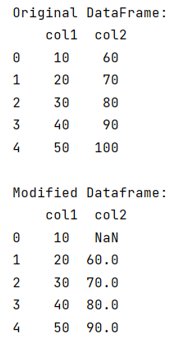# How to Shift a Column in Pandas Dataframe?

Given a Pandas DataFrame, we have to shift a column in it. By Pranit Sharma Last updated : September 23, 2023

## Shifting a Column in Pandas Dataframe

In pandas, we sometimes need to shift column or row values by some factor. This is allowed in pandas for better and more effective data analysis, pandas provide a method called the shift() method which helps shift a column by some factor. If we want the column values to be shifted by 1 value, we will pass the 1 inside shift() method or if we want to values to be shifted by N values, we will pass N inside shift().

Note

To work with pandas, we need to import pandas package first, below is the syntax:

```import pandas as pd
```

Let us understand with the help of an example,

## Python program to shift a column in Pandas Dataframe

```# Importing Pandas package
import pandas as pd

# Creating a dictionary
d = {
'col1':[10,20,30,40,50],
'col2':[60,70,80,90,100]
}

# Creating a DataFrame
df = pd.DataFrame(d)

# Display original DataFrame
print("Original DataFrame:\n",df,"\n")

# Shifting the col2 values by 1
df['col2'] = df.col2.shift(1)

# Display modified DataFrame
print("Modified Dataframe:\n",df)
```

### Output

The output of the above program is: